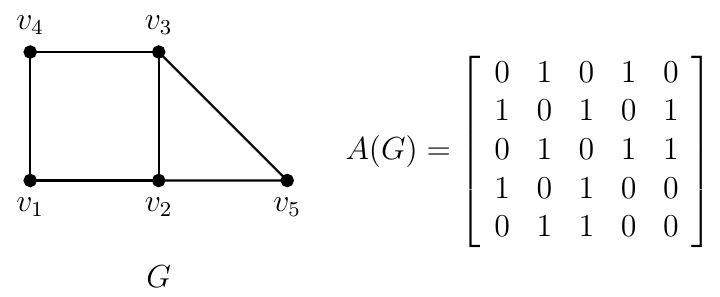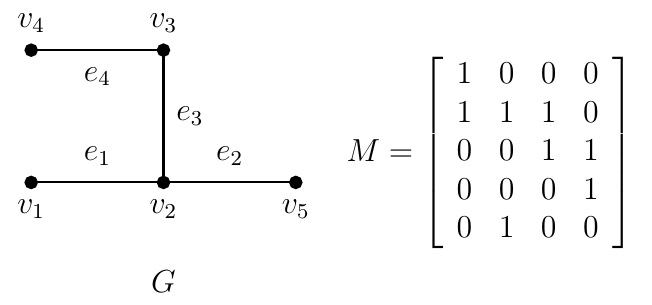Discrete Math Home

How to store information about a given graph $$G$$? Is there any alternative way to represent $$G$$ rather than writing its vertex set and edge set?

Definition (Adjacency matrix). Let $$G$$ be a simple graph on $$n$$ vertices $$v_1,v_2,\ldots,v_n$$. The adjacency matrix $$A=[a_{ij}]$$ of $$G$$ is the $$n\times n$$ binary matrix such that $a_{ij}=\begin{cases} 1 & \text{if } \{v_i,v_j\}\text{ is an edge in } G\\ 0 & \text{otherwise.} \end{cases}$ Sometimes it is denoted by $$A(G)$$ or $$A_G$$.

Example.Remark. If $$G$$ is a simple digraph, then the $$(i,j)$$-entry of its adjacency matrix $$A=[a_{ij}]$$ is $$1$$ if and only if $$(v_i,v_j)$$ is an arc in $$G$$.

Problem. What is the sum of entries in a row (column) of the adjacency matrix $$A$$ of a simple graph $$G$$? What if $$G$$ is a simple digraph?

Solution. If $$G$$ is a simple graph, then the sum of entries of row (column) $$i$$ of $$A$$ is $$\operatorname{d}(v_i)$$, the degree of the vertex $$v_i$$. If $$G$$ is a simple digraph, then the sum of entries of row $$i$$ of $$A$$ is the out-degree of the vertex $$v_i$$ and the sum of entries of column $$i$$ of $$A$$ is the in-degree of the vertex $$v_i$$.

Theorem. Let $$G$$ be a simple graph on $$n$$ vertices $$v_1,v_2,\ldots,v_n$$ with the adjacency matrix $$A$$. The number of walks of length $$k$$ from $$v_i$$ to $$v_j$$ is the $$(i,j)$$-th entry of $$A^k$$.

Note that the statement is true for $$k=1$$ because the $$(i,j)$$-entry of $$A$$ is $$1$$ if and only if there is an edge between $$v_i$$ and $$v_j$$. Prove by induction on $$k$$.

Example. For the adjacency matrix $$A$$ of the graph in the above example, $A^2=\left[\begin{array}{ccccc} 2&0&2&0&1\\ 0&3&1&2&1\\ 2&1&3&0&1\\ 0&2&0&2&1\\ 1&1&1&1&2 \end{array}\right],\; A^3=\left[\begin{array}{ccccc} 0&5&1&4&2\\ 5&2&6&1&4\\ 1&6&2&5&4\\ 4&1&5&0&2\\ 2&4&4&2&2 \end{array}\right].$ Note that $$(A^2)_{13}=2$$ and there are 2 walks of length $$2$$ from $$v_1$$ to $$v_3$$: $$v_1v_2v_3$$ and $$v_1v_4v_3$$. The diagonal entries of $$A^2$$ are the degrees of the vertices. Also $$(A^3)_{12}=5$$ and there are 5 walks of length $$3$$ from $$v_1$$ to $$v_2$$: $v_1v_4v_3v_2, v_1v_4v_1v_2, v_1v_2v_5v_2, v_1v_2v_3v_2, v_1v_2v_1v_2.$

Definition (Incidence matrix). Let $$G$$ be a simple graph with $$n$$ vertices $$v_1,v_2,\ldots,v_n$$ and $$m$$ edges $$e_1,e_2,\ldots,e_m$$. The incidence matrix $$M=[m_{ij}]$$ of $$G$$ is the $$n\times m$$ binary matrix such that $m_{ij}=\begin{cases} 1 & \text{if } e_j\text{ is incident with } v_i\\ 0 & \text{otherwise.} \end{cases}$ Example.Problem. What is the sum of entries in a row and column of the incidence matrix $$M$$ of a simple graph $$G$$?
Solution. If $$G$$ is a simple graph, then the sum of entries of row $$i$$ of $$M$$ is $$\operatorname{d}(v_i)$$, the degree of the vertex $$v_i$$ and the sum of entries of each column of $$M$$ is $$2$$.

Definition (Laplacian matrix). Let $$G$$ be a simple graph on $$n$$ vertices $$v_1,v_2,\ldots,v_n$$ with the adjacency matrix $$A$$. The degree matrix of $$G$$ is the $$n\times n$$ diagonal matrix $$D=[d_{ij}]$$ such that $$d_{ii}=\operatorname{d}(v_i)$$ for $$i=1,2,\ldots,n$$. The Laplacian matrix of $$G$$, denoted by $$L$$, is $$L=D-A$$. The signless Laplacian matrix of $$G$$, denoted by $$Q$$, is $$Q=D+A$$.

Example. For the above graph $$G$$, $D=\left[\begin{array}{ccccc} 1&0&0&0&0\\ 0&3&0&0&0\\ 0&0&2&0&0\\ 0&0&0&1&0\\ 0&0&0&0&1 \end{array}\right],\; A=\left[\begin{array}{ccccc} 0&1&0&0&0\\ 1&0&1&0&1\\ 0&1&0&1&0\\ 0&0&1&0&0\\ 0&1&0&0&0 \end{array}\right],$ $L=D-A=\left[\begin{array}{rrrrr} 1&-1&0&0&0\\ -1&3&-1&0&-1\\ 0&-1&2&-1&0\\ 0&0&-1&1&0\\ 0&-1&0&0&1 \end{array}\right],$ $Q=D+A=\left[\begin{array}{ccccc} 1&1&0&0&0\\ 1&3&1&0&1\\ 0&1&2&1&0\\ 0&0&1&1&0\\ 0&1&0&0&1 \end{array}\right].$
Observation. Let $$G$$ be a simple graph with the Laplacian Matrix $$L$$, signless Laplacian Matrix $$Q$$, and incidence matrix $$M$$.

1. The sum of each row of $$L$$ is $$0$$.

2. $$Q=MM^T$$.

3. $$L=NN^T$$ where $$N$$ is an oriented incidence matrix of $$G$$ obtained from $$M$$ by replacing one $$1$$ in each column of $$M$$ by $$-1$$ (i.e., making each edge of $$G$$ oriented).

Last edited